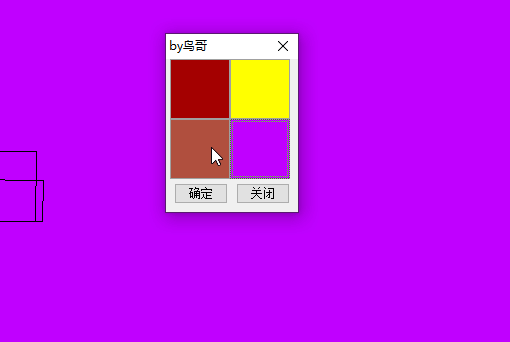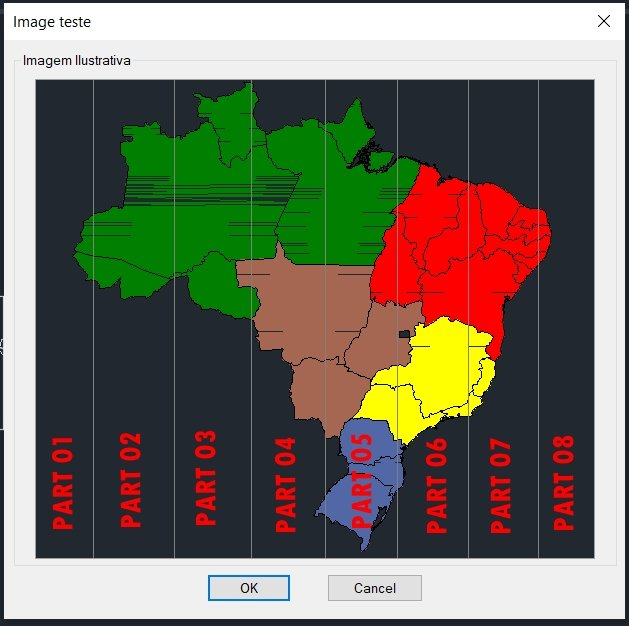# multiple actions or divide the image_button of DCL into equal parts## Recommended PostsPosted (edited)

Can someone give me an explanation of how I do it so that when I click on a certain part of image_button it will return me a viable of that part.

Something like thatLSP

```(defun c:sldteste ( )
(cond
( (<= (setq dcls (load_dialog "slidetest.dcl")) 0)
)
( (not (new_dialog "dcl_img_test" dcls "" (cond ( *screenpoint* ) ( '(-1 -1) ))))
)
(t
(setq w (dimx_tile "img_sld")
h (dimy_tile "img_sld")
)
(start_image "img_sld")
(fill_image 0 0 w h 0)
(slide_image -290 -440 (* w 2) (* h 2) "C:\\utilitarios\\mapabrasil2.sld")
(end_image)

(if (eq (start_dialog) 1)
(prompt "ok")
)
)
)
)```

DCL

```butt01 : button
{
width = 15; height = 2; fixed_width = true; alignment = centered;
}

dcl_img_test : dialog
{
label = "Image teste";
spacer;
: row
{
: boxed_column
{
label = "Imagem Ilustrativa";
width = 5;
: row
{
spacer;
: image_button
{
key = "img_sld";
width = 70; height = 30; color = 0; fixed_width=true; fixed_height=true; aspect_ratio = 1; alignment = centered;
}
spacer;
}
}
}
: row
{
ok_cancel;
}
spacer;
}```

Edited by Anushka
##### Share on other sitesHeres somewhat related topic.

•1
##### Share on other sitesAn example also some 3x3 & 4x4 dcl.

```;;;               Uses DD3X3.DCL for the dialogue definition.  The
;;;               slide images are in list ai_pts_lst.120

; ctone is slb library name (tlds) is slide name in library
; use individual slide name if no library
(setq ai_pts_lst '("ctone(TLDS)" "ctone(dsdn)" "ctone(trds)"
"ctone(dsr)" "ca3blank" "ctone(DSL)"
"ctone(blds)" "ctone(dsup)" "ctone(brds)")
)

(setq ai_pts_lst2 '("33sq1" "33sq2" "33sq3" "33sq4" "33sq5" "33sq6""33sq7" "33sq8" "33sq9" ))

(defun sq_pick ()
(cond
((= ans "33sq1")(setq ang1 4.7125)(setq ang2 0.0)(tr))
((= ans "33sq2")(tm))
((= ans "33sq3")(setq ang1 3.1417)(setq ang2 4.7125)(tr))
((= ans "33sq4")(tm))
((= ans "33sq5")(princ "run other clean up"))
((= ans "33sq6")(tm))
((= ans "33sq7")(setq ang1 1.5708)(setq ang2 0.0)(tr))
((= ans "33sq8")(tm))
((= ans "33sq9")(setq ang1 1.5708)(setq ang2 3.1417)(tr))
)
)

; calculates next slide
(defun alan4 ()
(setq x (+ x 1))
(setq sldname (nth x ai_pts_lst))
)

; third step
; set up slide libraray
(defun ai_ptype_start ()
(setq x -1)
(foreach pts0 ai_pts_lst2
(alan4)
(start_image pts0)
(slide_image 0 0 (- (dimx_tile pts0) 1) (- (dimy_tile pts0) 1) sldname)
(end_image)
)
)

; this is second step
(defun ai_ptype_main (/ globals)
(ai_ptype_start)
;now check each sq if picked then run alan2
(foreach pd0 ai_pts_lst2
(action_tile  pd0  "(done_dialog)(setq ans \$key)")
)
(start_dialog)

)

; this is first step

(if (= w2 nil)(setw2))

(setq app "dd3x3.dcl")
(if (= id_3x3 nil)
(progn
(setq id_3x3 dcl_id)
)
)
(if (not (new_dialog "dd3x3" id_3x3))
(exit))
(ai_ptype_main)
(sq_pick)
(setq *error* old_error old_error nil)

```

•1
##### Share on other sites16 hours ago, Grrr said:

Heres somewhat related topic.

Thanks @Grrr This worked perfectly!!!

##### Share on other sites1 hour ago, Anushka said:

Thanks @Grrr This worked perfectly!!!

I remember coding this out after I've got the answer in that topic.

## Join the conversation

You can post now and register later. If you have an account, sign in now to post with your account.
Note: Your post will require moderator approval before it will be visible.×   Pasted as rich text.   Restore formatting

Only 75 emoji are allowed.